# Rotational Work and Energy

## Rotational Motion of Class 11

The rotational work done by a force about the fixed axis of rotation is defined as

Wrot =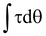(10.23)

Where τ is the torque produced by the force, and dθ is the infinitesimally small angular displacement about the axis.

The rotational kinetic energy of a body about a fixed rotational axis is defined as

Krot = 1/2 Iω2 (10.24)

where I is the moment of inertia about the axis.

Work – Energy Theorem

In complete analog to the work energy theorem for the translatory motion, it can be stated for rotational motion as:

Wrot = ΔKrot (10.25)

The net rotational work done by the forces is equal to the change in rotational kinetic energy of the body.

### Conservation of Mechanical Energy

In the absence of dissipative work done by non-conservative forces, the total mechanical energy of a system is conserved.

ΔK + ΔU = 0

or Kf + Uf = Ki + Ui (10.26)

Example 10.12

 A block of mass m = 4 kg is attached to a spring (k = 32 N/m) by a rope that hangs over a pulley of mass M = 8 kg. If the system starts from rest with the spring unextended, find the speed of the block after it falls 1m. Treat the pulley as a disc, so I = 1/2MR2 Solution Since the rim of the pulley moves at the same speed as the block, the speed of the block and the angular velocity of the pulley related by v = ωR.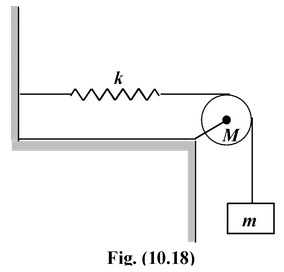When the block falls by a distance x, its potential energy decreases (ΔUg = -mgx); the potential energy of the spring increases (ΔUs = +1/2kx2), and both the block and the pulley gain kinetic energy (ΔK = 1/2mv2 + 1/2Iω2).

From the conservation of mechanical energy, ΔK + ΔU = 0,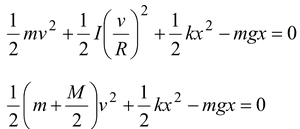Note that R was not needed.

Putting m = 4 kg; M = 8 kg; k = 32 N/m; x = 1m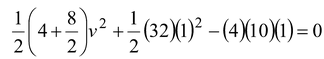4v2 + 16 – 40 = 0

orv = 2.4 m/s

### Rotational Power

In complete analog with the linear motion, the instantaneous rotational power is defined as

Prot =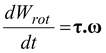(10.27)

 Example 10.13 A motor rotates a pulley of radius 25 cm at 20 rpm. A rope around the pulley lifts a 50 kg block, as shown in figure. What is the power output of the motor? Solution The tension in the rope is equal to the weight since there is no acceleration. Thus, T = 500 N.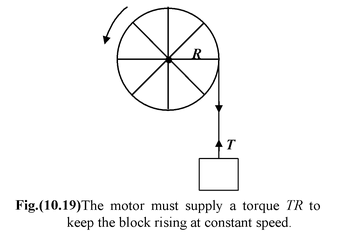Therefore, τ = TR = (500)(0.25) = 125 Nm.

Angular velocity, ω = 2πN/60

or ω = 2π(20)/60 = 2π/3 rad/s

The power required is

P = τω = (125 N m) = 2π/3 rad/s = 260 W